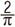# Shock Test Machine SelectionShock test machine selectionBasic definition：

● Acceleration of gravity
the accelerated velocity in the surface of earth caused by gravity.
● Pulse duration：
Shock pulse rises from the reference value to the maximum value.
● Severity of shock test
It’s the combination of peak acceleration and nominal pulse duration.
● Velocity variation
The absolute value of sudden velocity variation caused by the implementation of specified acceleration.
● Basic pulse waveform

a. Half-sine pulse: Ideal pulse with the time course curve which is half period of half-sine wave.
b. Postpeak sawtooth pulse: Ideal shock pulse with the time course curve which has asymmetric triangle with short falling time.
c. Trapezoidal pulse: Time course curve is the symmetric quadrangle with short rising and falling time.

Computational formula

⊙ Half-sine wave：v=AD×10-3

△v=Velocity variation, unit m/s; A=acceleration, unit m/s²;
D=Pulse duration, unit ms.

Model selection：

Velocity variation of different pneumatic shock and collision test machines under the conditions of no-load and nominal load are indicated on the samples in the show room, customer can find suitable machines according to their own requirements.

For example: The weight of test specimen is 30kg, size is: 400x400mm, need to meet the half-sine test index of 100g, 11ms, according to the below formula:
Half-sine wave：v=AD×10-3
=2×1000（m/s²）×11（ms）÷3.14÷1000